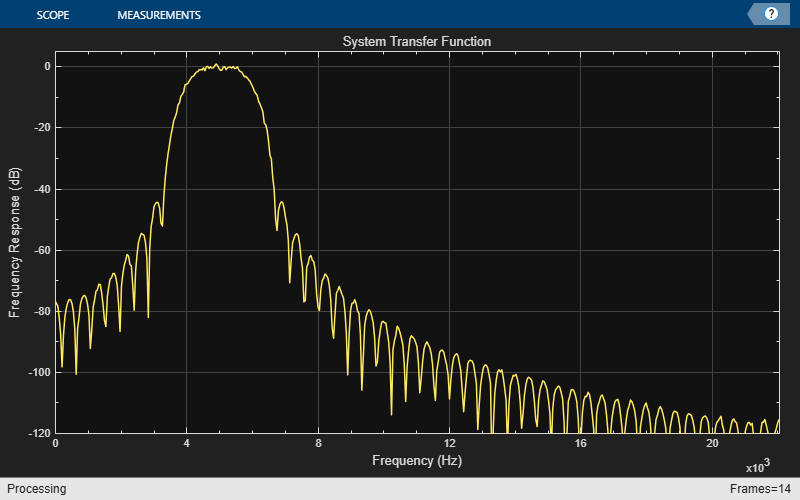Documentation

### This is machine translation

Mouseover text to see original. Click the button below to return to the English version of the page.

# dsp.VariableBandwidthFIRFilter

Variable bandwidth FIR filter

## Description

The `dsp.VariableBandwidthFIRFilter` object filters each channel of the input using FIR filter implementations. It does so while having the capability of tuning the bandwidth.

To filter each channel of the input:

1. Create the `dsp.VariableBandwidthFIRFilter` object and set its properties.

2. Call the object with arguments, as if it were a function.

## Creation

### Syntax

``vbw = dsp.VariableBandwidthFIRFilter``
``vbw = dsp.VariableBandwidthFIRFilter(Name,Value)``

### Description

````vbw = dsp.VariableBandwidthFIRFilter` returns a System object™, `vbw`, which independently filters each channel of the input over successive calls to the object. The filter’s cutoff frequency may be tuned during the filtering operation. The variable bandwidth FIR filter is designed using the window method.```

example

````vbw = dsp.VariableBandwidthFIRFilter(Name,Value)` returns a variable bandwidth FIR filter System object, `vbw`, with each property set to the specified value. You can specify additional name-value pair arguments in any order as (`Name1,Value1,...,NameN,ValueN`).```

## Properties

expand all

Unless otherwise indicated, properties are nontunable, which means you cannot change their values after calling the object. Objects lock when you call them, and the `release` function unlocks them.

If a property is tunable, you can change its value at any time.

Input sample rate, specified as a positive scalar in Hz. This property is non-tunable.

Data Types: `double` | `single`

Specify the type of the filter as one of ```'Lowpass' | 'Highpass' | 'Bandpass' | 'Bandstop'```. This property is non-tunable.

Specify the order of the FIR filter as a positive integer scalar. This property is non-tunable.

Data Types: `double` | `single`

Specify the window function used to design the FIR filter as one of `'Hann'` | `'Hamming'` | `'Chebyshev'` | `'Kaiser'`. This property is non-tunable.

Specify the Kaiser window parameter as a real scalar. This property is non-tunable.

#### Dependencies

This property applies when you set the `'Window'` property to `'Kaiser'`.

Data Types: `double` | `single`

Specify the filter cutoff frequency in Hz as a real, positive scalar, smaller than the `SampleRate/2`.

Tunable: Yes

#### Dependencies

This property applies if you set the `FilterType` property to `'Lowpass'` or `'Highpass'`.

Data Types: `double` | `single`

Specify the filter center frequency in Hz as a real, positive scalar, smaller than `SampleRate/2`.

Tunable: Yes

#### Dependencies

This property applies when you set the `FilterType` property to `'Bandpass'` or `'Bandstop'`.

Data Types: `double` | `single`

Specify the filter bandwidth in Hertz as a real, positive scalar, smaller than `SampleRate/2`.

Tunable: Yes

#### Dependencies

This property applies if you set the `FilterType` property to `'Bandpass'` or `'Bandstop'`.

Data Types: `double` | `single`

Specify the Chebyshev window attenuation as a real, positive scalar in decibels (dB). This property is non-tunable.

#### Dependencies

This property applies if you set the `Window` property to `'Chebyshev'`.

Data Types: `double` | `single`

## Usage

For versions earlier than R2016b, use the `step` function to run the System object algorithm. The arguments to `step` are the object you created, followed by the arguments shown in this section.

For example, `y = step(obj,x)` and `y = obj(x)` perform equivalent operations.

### Syntax

``y = vbw(x)``

### Description

example

````y = vbw(x)` filters the input signal `x` using the variable bandwidth FIR filter to produce the output `y`. The variable bandwidth FIR filter object operates on each channel, which means the object filters every column of the input signal independently over successive calls to the algorithm.```

### Input Arguments

expand all

Data input, specified as a vector or a matrix. This object also accepts variable-size inputs. Once the object is locked, you can change the size of each input channel, but you cannot change the number of channels.

Data Types: `double` | `single`
Complex Number Support: Yes

### Output Arguments

expand all

Filtered output, returned as a vector or a matrix. The size, data type, and complexity of the output signal matches that of the input signal.

Data Types: `double` | `single`
Complex Number Support: Yes

## Object Functions

To use an object function, specify the System object as the first input argument. For example, to release system resources of a System object named `obj`, use this syntax:

`release(obj)`

expand all

 `freqz` Frequency response of filter `fvtool` Visualize frequency response of DSP filters `impz` Impulse response of discrete-time filter System object `info` Information about filter System object `coeffs` Filter coefficients `cost` Estimate cost for implementing filter System objects `grpdelay` Group delay response of discrete-time filter System object
 `step` Run System object algorithm `release` Release resources and allow changes to System object property values and input characteristics `reset` Reset internal states of System object

## Examples

expand all

Note: This example runs only in R2016b or later. If you are using an earlier release, replace each call to the function with the equivalent `step` syntax. For example, myObject(x) becomes step(myObject,x).

This example shows you how to tune the center frequency and the bandwidth of the FIR filter.

```Fs = 44100; % Input sample rate % Define a bandpass variable bandwidth FIR filter: vbw = dsp.VariableBandwidthFIRFilter('FilterType','Bandpass',... 'FilterOrder',100,... 'SampleRate',Fs,... 'CenterFrequency',1e4,... 'Bandwidth',4e3); tfe = dsp.TransferFunctionEstimator('FrequencyRange','onesided'); aplot = dsp.ArrayPlot('PlotType','Line',... 'XOffset',0,... 'YLimits',[-120 5], ... 'SampleIncrement', 44100/1024,... 'YLabel','Frequency Response (dB)',... 'XLabel','Frequency (Hz)',... 'Title','System Transfer Function'); FrameLength = 1024; sine = dsp.SineWave('SamplesPerFrame',FrameLength); for i=1:500 % Generate input x = sine() + randn(FrameLength,1); % Pass input through the filter y = vbw(x); % Transfer function estimation h = tfe(x,y); % Plot transfer function aplot(20*log10(abs(h))) % Tune bandwidth and center frequency of the FIR filter if (i==250) vbw.CenterFrequency = 5000; vbw.Bandwidth = 2000; end end ```## Algorithms

expand all

 Jarske, P.,Y. Neuvo, and S. K. Mitra, "A simple approach to the design of linear phase FIR digital filters with variable characteristics." Signal Processing. Vol. 14, Issue 4, June 1988, pp. 313-326.

Watch now